• 存储管理中页面置换算法性能测试 要求：设系统采用固定分配局部置换的存储分配策略，编写仿真程序对下述页面 置换算法进行性能测试，并对结果进行分析和比较。 (1) 最佳适应(Optimal)页面置换算法； (2) 先进先...LRU
• LRU页面置换算法C++代码 常见的缓存算法： LRU：(least recently used) 最近最少使用，思想是如果一个数据刚被访问过，那么将来被访问的几率也更高 LFU：(least frequently used) 最少经常使用，思想是如果一个...
LRU页面置换算法C++代码

常见的缓存算法：

LRU：(least recently used) 最近最少使用，思想是如果一个数据刚被访问过，那么将来被访问的几率也更高
LFU：(least frequently used) 最少经常使用，思想是如果一个数据很久不被访问，那么将来被访问的几率也不大
FIFO：(first in first out) 先进先出，思想是如果一个数据最先进入缓存，则应该最早淘汰掉
我们这里主要看一看LRU缓存的规则：

新数据插入到链接头部
每当数据被访问，则将数据移到链表头部
当链表满的时候，则将链表尾部的数据删除，在将新数据插入链表头部

LRU：cache应该具备的两个操作：

get（key）：如果key在hashmap中存在，则直接查找其在list链表中的位置，然后将其移动到链表头部再更新其hashmap的映射
set（key，value）：如果key在hashmap中不存在，并且页面还满了，则删除尾部节点，再插入，如果存在于hashmap中，则将其从list链表中移动到链表头部，再更新其hashmap的映射
我们现在自己手动实现一下：

我们这里LRU使用的是list + map来实现，采用list的原因是可以快速移动数据，而map用来保存节点的key，value的值，便于能快速根据key值找点其在list中对应的位置，所以value值保存的是list的迭代器类型

list + map的代码如下：

//查找时间复杂度 是map 红黑树 logn
#include <iostream>
#include <vector>
#include <list>
#include <map>
#include <hash_map>
using namespace std;

struct MyLRUnode
{
int key;//形成key_value结构，通过这个唯一的键 直接可以找到这个值
int value;
MyLRUnode(int a, int b):key(a), value(b){}
};

class LRUCache
{
public:
LRUCache(int size)
{
Lru_capacity = size;
}
int get(int key) //访问键为key的页面 找到 则将其重新插入到链表头
{
if(mymap.find(key) != mymap.end())//如果找到了
{
mylist.splice(mylist.begin(), mylist, mymap[key]);//则将mylist链表上找到的的iterator位置的元素重新链接到mylist.begin()位置上
mymap[key] = mylist.begin();//然后将map映射表中 键为key的页面映射位置重置一下
return mymap[key]->value;//最后返回将key对应的value返回
}
else//没找到 则返回-1
{
return -1;
}
}

void put(int key, int value)//访问key value页面是否存在，若存在则提前“并更新value值”，不存在则创建之后再提前
{
if(mymap.find(key) == mymap.end())//key值不在页中
{
if(mylist.size() == Lru_capacity)//不在页中并且页还满了
{
mymap.erase(mylist.back().key);//将list尾部节点对应的key和其页映射关系删除
mylist.pop_back();//将list尾部节点删除
}
MyLRUnode pnewnode(key, value);//创建新页
mylist.push_front(pnewnode);//插入页链表中
mymap[key] = mylist.begin();//key对应页面关系存入map
}
else//key值在页中
{
mymap[key]->value = value;//更新key对应的value值
mylist.splice(mylist.begin(), mylist, mymap[key]);//将mylist链表上找到的的iterator位置的元素重新链接到mylist.begin()位置上
mymap[key] = mylist.begin();//然后将map映射表中 键为key的页面映射位置重置一下
}
}

void show_list()
{
list<MyLRUnode>::iterator it = mylist.begin();
for(;it != mylist.end();)
{
cout << it->key << ":" << it->value <<endl;
it++;
}
cout << endl;
}
void show_map()
{
map<int, list<MyLRUnode>::iterator>::iterator it = mymap.begin();
while(it != mymap.end())
{
cout << it->first << ":" << (it->second)->value << endl;
it++;
}
cout << endl;
}
private:
int Lru_capacity;
list<MyLRUnode> mylist;
map<int, list<MyLRUnode>::iterator> mymap;//用来通过key键值 直接找到对应的双向链表节点
//hash_map<int, list<MyLRUnode>::iterator> myhash_map;
};

int main()
{
LRUCache lru(3);
lru.put(1,100);
lru.show_list();
lru.show_map();
lru.put(2,200);
lru.show_list();
lru.show_map();
lru.put(3,300);
lru.show_list();
lru.show_map();
lru.get(2);
lru.show_list();
lru.show_map();
lru.put(4,400);
lru.show_list();
lru.show_map();
return 0;
}


如果现在我们需要对其进行改进，map查找的时间复杂度还是有点大，是O(logn)，我们怎么对其进行修改，修改为时间复杂度为O(1)呢？

说到O(1)，我们第一时间想到的是哈希，所以我们可以使用hash_map将map替换掉即可，底层的hash_map会根据传入的key值，通过hash函数建立哈希表，以达到查找时间为O(1)

list + hash_map的代码如下：

//查找时间复杂度 是hash_map 映射表 查找时间复杂度 O(1)
#include <iostream>
#include <vector>
#include <list>
#include <map>
#include <hash_map>
using namespace std;

struct MyLRUnode
{
int key;//形成key_value结构，通过这个唯一的键 直接可以找到这个值
int value;
MyLRUnode(int a, int b):key(a), value(b){}
};

class LRUCache
{
public:
LRUCache(int size)
{
Lru_capacity = size;
}
int get(int key) //访问键为key的页面 找到 则将其重新插入到链表头
{
if(myhash_map.find(key) != myhash_map.end())//如果找到了
{
mylist.splice(mylist.begin(), mylist, myhash_map[key]);//则将mylist链表上找到的的iterator位置的元素重新链接到mylist.begin()位置上
myhash_map[key] = mylist.begin();//然后将map映射表中 键为key的页面映射位置重置一下
return myhash_map[key]->value;//最后返回将key对应的value返回
}
else//没找到 则返回-1
{
return -1;
}
}

void put(int key, int value)//访问key value页面是否存在，若存在则提前“并更新value值”，不存在则创建之后再提前
{
if(myhash_map.find(key) == myhash_map.end())//key值不在页中
{
if(mylist.size() == Lru_capacity)//不在页中并且页还满了
{
myhash_map.erase(mylist.back().key);//将list尾部节点对应的key和其页映射关系删除
mylist.pop_back();//将list尾部节点删除
}
MyLRUnode pnewnode(key, value);//创建新页
mylist.push_front(pnewnode);//插入页链表中
myhash_map[key] = mylist.begin();//key对应页面关系存入map
}
else//key值在页中
{
myhash_map[key]->value = value;//更新key对应的value值
mylist.splice(mylist.begin(), mylist, myhash_map[key]);//将mylist链表上找到的的iterator位置的元素重新链接到mylist.begin()位置上
myhash_map[key] = mylist.begin();//然后将map映射表中 键为key的页面映射位置重置一下
}
}

void show_list()
{
list<MyLRUnode>::iterator it = mylist.begin();
for(;it != mylist.end();)
{
cout << it->key << ":" << it->value <<endl;
it++;
}
cout << endl;
}
void show_map()
{
hash_map<int, list<MyLRUnode>::iterator>::iterator it = myhash_map.begin();
while(it != myhash_map.end())
{
cout << it->first << ":" << (it->second)->value << endl;
it++;
}
cout << endl;
}
private:
int Lru_capacity;
list<MyLRUnode> mylist;
//map<int, list<MyLRUnode>::iterator> mymap;//用来通过key键值 直接找到对应的双向链表节点
hash_map<int, list<MyLRUnode>::iterator> myhash_map;
};

int main()
{
LRUCache lru(3);
lru.put(1,100);
lru.show_list();
lru.show_map();
lru.put(2,200);
lru.show_list();
lru.show_map();
lru.put(3,300);
lru.show_list();
lru.show_map();
lru.get(2);
lru.show_list();
lru.show_map();
lru.put(4,400);
lru.show_list();
lru.show_map();
return 0;
}


展开全文• fifo&lru;页面置换算法c++实现，从trace.txt文档中读入置换页面序号fifo lru
• 页面置换算法页面置换算法页面置换算法页面置换算法
• 操作系统课程FiFO，OPT，LRU三种页面置换算法C++实现，代码清晰，有少量注释，希望给有上机的孩子们一些参考
• 页面置换算法源码 包含 opt lru fifo c++源码
• 操作系统页面置换算法 页面置换算法： 功能：选择被置换的物理页面 目标：尽可能减少页面的调入调出次数 把未来不再访问或者短期内不访问的页面调出 页面锁定：（以下部分） 描述必须常驻内存的逻辑页面...
操作系统页面置换算法

操作系统页面置换算法简单的c实现缺页率算法

页面置换算法：
功能：选择被置换的物理页面
目标：尽可能减少页面的调入调出次数
把未来不再访问或者短期内不访问的页面调出
页面锁定：（以下部分）
描述必须常驻内存的逻辑页面，操作系统的关键部分，要求响应速度的代码和数据，页表中的锁定标志位

置换算法的评价：
记录所有存储单元的轨迹。模拟置换算法，缺页率。

局部置换算法：
仅限于当前进程占用的物理页面
最优算法（预测未来，没法实现）：置换未来最长时间不使用的页面，
特征：缺页最少，实际系统无法实现，无法预知在每隔页面在下次访问前的等待时间。
作为其他算法的评价依据。

先进先出（FIFO），最近最久未使用算（过去使用，对未来的预测）法,近来与出去之间是否会被使用
时钟算法，最不常使用算法
选择在内存驻留时间最长的页面进行置换
实现：维护一个记录所有位于内存中的逻辑页面链表
链表元素按驻留内存的时间排序，
出现缺页时，进行链首去页面置换。
特征：
实现简单
性能较差，调出的页面可能是经常访问的
很少单独使用

最近最久未使用算法（LRU）：统计使用时间  ——–表面优秀算法，开销比较大
实现：缺页时，记录内存中每个逻辑页面的使用时间，选择最长时间未使用的页面进行置换。
实现算法：
1.链表维护，链首刚刚使用的页面，链尾最久未使用的页面
2.栈维护
以下对LRU的改进：

时钟页面置换算法（CLOCK）：
1.对页面的访问情况大致访问。
1.数据结构：在页表中增加访问位，描述在过去的一段时间内访问的情况
各个页面组织成环形链表
指针指向最先调入的页面
特征在LRU和FIFO折中。
页面装入内存时，访问位(存在页表项，被CPU调整)初始化为0
访问页面时，访问位位置1
缺页时，从指针当前位置顺序查找环形链表，访问位为0，则置换页面

改进的时钟算法：
增加修改位

最不常使用算法：（LFU）：统计使用次数
思路：缺页时，置换访问次数最少的页面
实现：每个页面增加一个访问计数
没有考虑程序运行的阶段问题。

随着分配的物理页面数 的增加，缺页的次数反而升高
原因：FIFO算法的置换特征与进程访问内存的动态特征矛盾
被它置换出去的页面并不一定是进程近期不会访问的

全局页面置换算法：给进程分配可变的物理页面
置换页面的选择范围是可能换出的页面
工作集置换算法：
进程当前正在使用的逻辑页面集合：W（t,s）t为时间窗口的大小
常驻集：在一段时间内内存中的页， 和工作集是交集，理想情况下工作集等价于常驻集，实际情况访问的页不再常驻集（内存中）。
思路：换出不再工作集的页面 （并不一定在缺页的时候），开销很大

缺页率算法（PFF）：
缺页次数/内存访问次数 或 缺页的平均时间间隔的倒数（常使用）
影响缺页率的因素：
页面置换算法、分配给进程的物理页面数目、页面的大小、程序的编写方法
通过调整常驻集的大小，使得每个进程的缺页率保持在一定范围内
1.  如果缺页率过高，则增加常驻集分配更多的页面
2.  如果缺页率过低，则减少常驻集分配更多的页面
做法：
访存设置引用位标志

抖动和负载控制：
进程物理页面太少，不能包含工作集
造成大量的缺页，频繁置换
进程运行速度变化

操作系统需要在并发和缺页率之间达到一个平衡

简单的c++实现缺页率算法

缺页率置换

#include<iostream>
#include<cmath>
#include<cstdlib>
#include<stdlib.h>
#include<string.h>
#include<set>
#include<vector>
using namespace std;

#define  MEM_SIZE 10
//此处内存动态分配逻辑物理页面的大小
int main()
{
int n=-1;
int t=4;
cout<<"-------------缺页率------------"<<endl;
cout<<"请输入程序需要的页号："<<endl;

//cout<<">>:输入0 选择缺页率模式";
vector<int>mem;

bool find=false;
int t1=0;
int t2=-1;
set<int>myset;
while(cin>>n)
{
cout<<n<<endl;
myset.insert(n);
t2++;
find=false;
for( vector<int>::iterator i=mem.begin();i!=mem.end();i++)
{
if(n==*i)
{

cout<<"内存中存在:"<<n<<endl;
find =true;
}
}

if (find) continue;

int aa[MEM_SIZE];
for(int i=0;i<MEM_SIZE;i++) aa[i]=-1;
int r=0;
if((t2-t1)>t)
{
t1=t2;
for(set<int>::iterator it=myset.begin();it!=myset.end();it++)
{

aa[r]=*it;
r++;
if(r>=MEM_SIZE) r=0;

}
myset.clear();
int rr=-1;
for( vector<int>::iterator i=mem.begin();i!=mem.end();i++)
{     bool findmem=false;
rr++;
for(int j=0;j<MEM_SIZE;j++)
{
if(*i==aa[j])
{
findmem=true;
break;
}

}
if(!findmem) mem.erase(i);

}
for(int i=0;i<MEM_SIZE;i++)
{
if(mem[i]==-1)
{
mem[i]=n;
}
}
}

}
return 0;
}
展开全文操作系统 内存
• 用一种计算机高级语言来实现请求分页存储管理方式先进先出（FIFO）置换算法，设计要求如下： ⑴ 能够输入给作业分配的内存块数； ⑵ 能够输入给定的页面，并计算发生缺页的次数以及缺页率； ⑶ 缺页时，如果发生...
• 计算并输出下述各种算法在不同内存容量下的命中率。 A. FIFO先进先出的算法 B. LRR最近最少使用算法 C. OPT最佳淘汰算法（先淘汰最不常用的页地址）
• 1、首先建立本次实验的流程图： 1、 然后建立储存相应...其中name表示页面名，nomber在lru算法中起到标记时间的作用 2、 设置一个指针，在fifo算法中指向最老进程 Physicalblock *p=pb;//指向最老进程的指针 3、...
1、首先建立本次实验的流程图：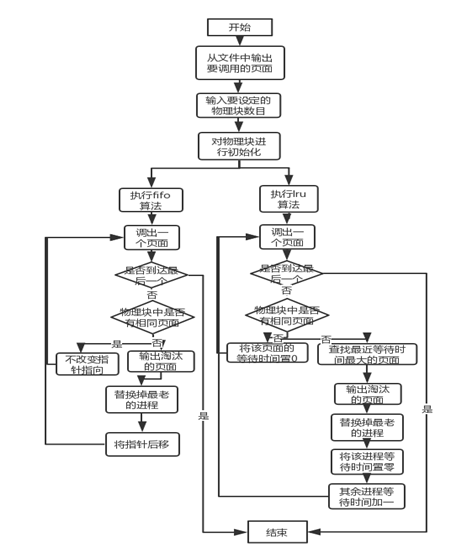1、	然后建立储存相应页面的物理块：
struct Physicalblock{
int name;
int nomber;//优先级1，2，3
}pb;

其中name表示页面名，nomber在lru算法中起到标记时间的作用
2、	设置一个指针，在fifo算法中指向最老进程
Physicalblock *p=pb;//指向最老进程的指针

3、	因为本程序中需要从文件中进行输出，所以本次实验在源文件夹中设置“data.txt”文件，储存需要用到的页面
执行程序如下：
ifstream openfile("data.txt");

4、	在程序开始时对进程块进行初始化：
	ifstream openfile2("data.txt");
while(n1--)
{
if(openfile2.eof())
{
cout<<"引用串已经分配完毕"<<endl;
break;
}
openfile2>>num;
pb[t].nomber=0;
pb[t++].name=num;
}
openfile2.close();

5、	调用fifo算法，在调用时，要先比对是否已经存在相同页面
如果有那么要标记一下，如果没有就将最老的替换掉，并且输出，输出之后将指向最老进程的指针后移
void fifo(int n1)//先进先出置换算法
{
cout<<"使用fifo的置换方法结果为："<<endl<<"淘汰的为：";
int num,t=1,flag=0,total=0;
ifstream openfile("data.txt");
while(!openfile.eof())
{
flag=0;
openfile>>num;
t++;
if(t>=n1)
{
for(int i=0;i<n;i++)
{
if(num==pb[i].name)
{
flag=1;
}
}
if(flag==0)
{
total++;
cout<<p->name<<"  ";
p->name=num;
p++;
if(total%n1==0)
{
p=pb;
}
}
}
}
openfile.close();
cout<<endl<<"缺页的总次数为："<<total<<endl;
cout<<"缺页率为： "<<(total/(s+0.0))*100<<"%"<<endl<<endl;
}

6、	在lru算法中要设置nomber用以记录每个进程等待的时间，每次替换之前要查找最大的，然后替换一个之后，这个页面的时间就要变为0，其他的要加一
void lru(int n1)//最近久未使用算法
{
cout<<"使用lru的置换方法结果为："<<endl<<"淘汰的为：";
int num,t=0,flag=0,total=0,count=0;
ifstream openfile("data.txt");
while(!openfile.eof())
{
int max=-999,maxnomber=0,flag=0;//flag=0表示没有一样的，=1表示有一样的
openfile>>num;
count++;
if(count>=n1)
{
for(int i=0;i<n1;i++)//找出最大的
{
if(num==pb[i].name)//检查是否有一样的
{
flag=1;//flag变为1
pb[i].nomber=0;//一样的这个变为0
for(int j=0;j<n1;j++)//其他几个的等待时间加一
{
if(i==j)//除了这个一样的 不用加一
{
continue;
}
else pb[j].nomber++;//其余的都要加一
}
}
if(pb[i].nomber>max)//同时再查找最大的
{
max=pb[i].nomber;
maxnomber=i;//maxnomber标记最大的序号
}
}
if(flag==0)//如果没有一样的
{
cout<<pb[maxnomber].name<<" ";//把淘汰的输出
total++;//总数加一
pb[maxnomber].name=num;//把淘汰的名字换掉
pb[maxnomber].nomber=0;//时间变为0
for(int j=0;j<n1;j++)//除了这个其他的都加一
{
if(j==maxnomber)	continue;
else pb[j].nomber++;
}
}
}
}
openfile.close();
cout<<endl<<"缺页的总次数为："<<total<<endl;
cout<<"缺页率为： "<<(total/(s+0.0))*100<<"%"<<endl;
}

7、	程序源代码如下：
#include<iostream>
#include<fstream>//进行文件读取的库函数
using namespace std;
int n,s=0;//n物理块数目,s页面总数
struct Physicalblock{
int name;
int nomber;//优先级1，2，3
}pb;
Physicalblock *p=pb;//指向最老进程的指针
void fifo(int n1)//先进先出置换算法
{
cout<<"使用fifo的置换方法结果为："<<endl<<"淘汰的为：";
int num,t=1,flag=0,total=0;
ifstream openfile("data.txt");
while(!openfile.eof())
{
flag=0;
openfile>>num;
t++;
if(t>=n1)
{	for(int i=0;i<n;i++)
{	if(num==pb[i].name)
{	flag=1;		}	}
if(flag==0)
{	total++;
cout<<p->name<<"  ";
p->name=num;
p++;
if(total%n1==0)
{p=pb;}}}}
openfile.close();
cout<<endl<<"缺页的总次数为："<<total<<endl;
cout<<"缺页率为： "<<(total/(s+0.0))*100<<"%"<<endl<<endl;
}
void lru(int n1)//最近久未使用算法
{
cout<<"使用lru的置换方法结果为："<<endl<<"淘汰的为：";
int num,t=0,flag=0,total=0,count=0;
ifstream openfile("data.txt");
while(!openfile.eof())
{
int max=-999,maxnomber=0,flag=0;//flag=0表示没有一样的，=1表示有一样的
openfile>>num;
count++;
if(count>=n1)
{ for(int i=0;i<n1;i++)//找出最大的
{if(num==pb[i].name)//检查是否有一样的
{flag=1;//flag变为1
pb[i].nomber=0;//一样的这个变为0
for(int j=0;j<n1;j++)//其他几个的等待时间加一
{	if(i==j)//除了这个一样的 不用加一
{continue;}
else pb[j].nomber++;//其余的都要加一
} }
if(pb[i].nomber>max)//同时再查找最大的
{max=pb[i].nomber;
maxnomber=i;//maxnomber标记最大的序号
}	}
if(flag==0)//如果没有一样的
{cout<<pb[maxnomber].name<<" ";//把淘汰的输出
total++;//总数加一
pb[maxnomber].name=num;//把淘汰的名字换掉
pb[maxnomber].nomber=0;//时间变为0
for(int j=0;j<n1;j++)//除了这个其他的都加一
{if(j==maxnomber)	continue;
else pb[j].nomber++;}} }}
openfile.close();
cout<<endl<<"缺页的总次数为："<<total<<endl;
cout<<"缺页率为： "<<(total/(s+0.0))*100<<"%"<<endl; }
int main()
{
ifstream openfile("data.txt");//打开分页序列
int num,n1,t=0,nomber=1;//num表示数字，n1用来临时取代n
cout<<"系统需要调用的页面有："<<endl;
while(!openfile.eof())
{s++;
openfile>>num;
cout<<num<<"  "; }
cout<<endl;
openfile.close();
cout<<"请输入物理块的个数："<<endl;
cin>>n;	n1=n;
ifstream openfile2("data.txt");
while(n1--)
{if(openfile2.eof())
{cout<<"引用串已经分配完毕"<<endl;
break;}
openfile2>>num;
pb[t].nomber=0;
pb[t++].name=num;}
openfile2.close();
fifo(n);
lru(n);}

IV.	分析和讨论
本次实验输入3个物理块，实验数据为：
7 0 1 2 0 3 0 4 2 3 0 3 2 1 2 0 1 7 0 1
结果如图所示：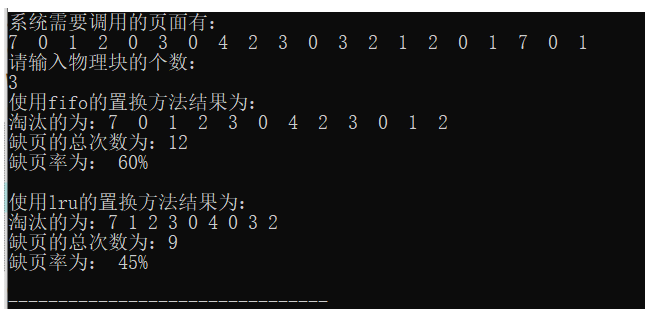所以可以看出fifo算法的缺页率是比较高的，fifo会使一些页面频繁的被替换掉，导致缺页率较高，二而lru算法缺页率是比较低的，效率要好一些
V.	结论
Fifo算法的原理就是将最先进来hiy的给替换掉，不考虑进程频繁切换进程的后果，lru算法的原理是将最近一段时间内没有访问到的进程给替换掉，也就是lru只考虑最久没有被访问的页面将其替换掉这是两种思路。Fifo算法的缺点很显而易见就是因为它总是优先把先进来的替换掉，如果在一个程序里面交替出现了很多次这样的页面，那么这个页面会不断地把前面的顶替掉因此会出现很多缺页，而lru算法就可以避免这个问题。本次编程时fifo算法相对比较简单，因为只需要设置一个指针，每次往后指就好了，而写lru算法的时候计算每个进程的等待时间是一个挺麻烦的事情，一个不小心就导致全部出错，另外本次要从文件中读取，有好几次从文件中读取之后忘记关闭文件了，也造成了很大的问题。


展开全文操作系统
• 操作系统实验 页面置换算法 c++程序代码 缺页次数 缺页率 物理块 FIFO置换算法 LRU置换算法 最佳置换算法
• 本次我要写的文章是操作系统的页面置换算法（OPT、FIFO、LRU、CLOCK）。页面置换算法不是很难，想必搜我这种文章的应该是都懂原理。所以在这里，简单概括算法特点，主要是展示代码。最佳置换算法——OPT（Optimal）...人在美国，刚下飞机！小白第一次写知乎文章，如果有不准确的地方，请各位大神多多指教。本次我要写的文章是操作系统的页面置换算法（OPT、FIFO、LRU、CLOCK）。页面置换算法不是很难，想必搜我这种文章的应该是都懂原理。所以在这里，简单概括算法特点，主要是展示代码。最佳置换算法——OPT（Optimal）置换算法OPT是一种理想的置换算法。当要调入一页面而必须淘汰一个旧页面时，所淘汰的页面应该是以后不再访问的或距现在最长时间后再访问的页面，该置换算法的缺页中断率最低。根据其原理，我们不难发现OPT是需要知道现有作业的页面访问序列的，而实际情况中，我们不可能知道页面的访问序列的，因此OPT在实际中不可能实现，但我们常把它作为衡量其他置换算法效率的标准。先进先出置换算法——FIFO（First In First Out）置换算法FIFO算法总是淘汰最先进入主存的页面，该算法比较简单，但算法效率不高。最近最久未用置换算法——LRU（Least Recently Used）置换算法LRU算法总是淘汰最后一次访问时间距当前时间间隔最长的页面。该算法是一种通用算法，因而广泛被采用。时钟（CLOCK）置换算法该算法是采用环形链表表示进程所访问的页面（模仿圆形时钟），引入一个指针指向最早进入主存的页面（就像时钟指针指向某个时间一样），这样只需移动指针来查找满足条件的页面。首先，给每个进入页表的页面设置一个“访问位”，对于刚刚进入页表的页面“访问位”设置为“1”。每次发生缺页中断时，若指针当前指向的页面访问位为“0”，就将当前页面置换出去，并且指针从当前指向的页面改为指向页表中的下一个页面。若指针当前指向的页面访问位为“1”，那么就将该页面访问位从“1”改为“0”。若不发生缺页中断，则指针不移动，并且将需要访问页面在页表中的“访问号”改为“1”。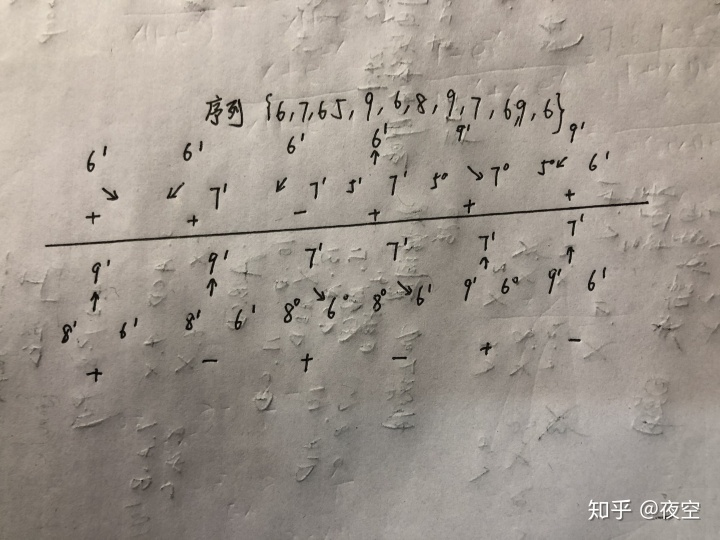代码#include<iostream>
#include<vector>
#include<map>
#include<algorithm>

using namespace std;
class PRA
{
public:
int row;  //页表中共有row个物理块
int column;  //页面访问序列长度
int *QString;  //声明一个指针
vector<vector<int>> PageTable; // 存储历史上所有的出现的页表
public:
PRA(int row,int column,int a[]);//  构造函数
tuple<vector<vector<int>>,int> OPT(void);
tuple<vector<vector<int>>,int> FIFO(void);
tuple<vector<vector<int>>,int> LRU(void);
tuple<vector<vector<int>>,int> CLOCK(void);
void Print(vector<vector<int>> b,int BreakPage);
};
// 初始化类
PRA::PRA(int row,int column,int a[])
{
this->row = row;
this->column = column;
QString = a;
}

tuple<vector<vector<int>>,int> PRA::OPT(void)
{
int position;
int max_value=0,max_key=0;
map<int,int> distince;  // 用来存储页表中页面距再次访问该页面的序列间隔
vector<int> block;  // 用来表示当前页表中的页面页号
int i = 1,counter = 0;  // i用来跟踪需访问的页号，counter记录缺页次数
PageTable.clear();  // PageTable用来存储历史上所有的页表
block.push_back(QString[i-1]); // 页表为空时，直接把待访问的页面直接装入就好啦
PageTable.push_back(block); // 把当前页表保存到存储历史上所有页表的容器中
counter++;  //  由于页表装入新的页面，缺页次数+1
block.clear();  //  清除当前页表，以便下一次页表存放所有的页面
//  页表还没有放满的情况
while(PageTable[i-1].size()<3 && i<this->column)
{
// 查看是否发生缺页中断
vector<int>::iterator ret;
ret = find(PageTable[i-1].begin(), PageTable[i-1].end(), QString[i]);
if(ret != PageTable[i-1].end())  // 没有发生缺页中断
{
for(int j=0;j < PageTable[i-1].size();j++)
block.push_back(PageTable[i-1][j]);
PageTable.push_back(block);
block.clear();
i++;
}
else  // 发生缺页中断
{
for(int j=0;j < PageTable[i-1].size();j++)
block.push_back(PageTable[i-1][j]);
block.push_back(QString[i]);
PageTable.push_back(block);
block.clear();
counter++;
i++;
}
}
while(i < this->column)  // 页表已经放满
{
vector<int>::iterator ret;
ret = find(PageTable[i-1].begin(), PageTable[i-1].end(), QString[i]);
if(ret != PageTable[i-1].end())  // 没有发生缺页中断
{
for(int j=0;j < PageTable[i-1].size();j++)
block.push_back(PageTable[i-1][j]);
PageTable.push_back(block);
block.clear();
i++;
}
else  //  发生了缺页中断
{
counter++;
max_value = 0;
for(int j=0;j < PageTable[i-1].size();j++)
{
for(position=i+1;position<this->column;position++) //  求前一次页表中所有页面与再次访问该页面的序列间隔，以便确定缺页时，置换出哪一个页面
{
if(PageTable[i-1][j] == QString[position])
{
distince[PageTable[i-1][j]] = position-i+1;
// 记录存储页表中页面距再次访问该页面的序列间隔
break;
}
else
{
distince[PageTable[i-1][j]] = this->column;
//  前一次页表中的页面后续不会再次访问，就将其序列间隔设置为整个页面访问序列的长度，这样使得不再被访问的页面必定能够置换出去
}
}
if(distince[PageTable[i-1][j]] > max_value) //  求出前一次页表中页面再次被访问的最大序列间隔值
max_value = distince[PageTable[i-1][j]];
}
//找出距现在最长时间后再访问的页面
auto find_item = find_if(distince.begin(), distince.end(),[max_value](const map<int, int>::value_type item)
{
return item.second == max_value;
});
if (find_item!= distince.end())  //  找出最大间隔值对应的页面
{
max_key = (*find_item).first;
}
distince.clear();

for(int j=0;j < PageTable[i-1].size();j++)
{
if(PageTable[i-1][j]==max_key)
block.push_back(QString[i]);
else
block.push_back(PageTable[i-1][j]);
}
PageTable.push_back(block);
block.clear();
i++;
}
}

return make_tuple(PageTable,counter);
}

//  FIFO实现很简单，就像队列一样，若发生缺页中断每次把最先进入页表中的页面置换出去，若不发生缺页中断当前页表就复制前一次页表中的页面就可以了tuple<vector<vector<int>>,int> PRA::FIFO(void)
{
vector<int> block;
PageTable.clear();
int i = 1,counter = 0;
block.push_back(QString[i-1]);
PageTable.push_back(block);
counter++;
block.clear();
while(PageTable[i-1].size()<3 && i<this->column)
{
vector<int>::iterator ret;
ret = find(PageTable[i-1].begin(), PageTable[i-1].end(), QString[i]);
if(ret != PageTable[i-1].end())
{
for(int j=0;j < PageTable[i-1].size();j++)
block.push_back(PageTable[i-1][j]);
PageTable.push_back(block);
block.clear();
i++;
}
else
{
for(int j=0;j < PageTable[i-1].size();j++)
block.push_back(PageTable[i-1][j]);
block.push_back(QString[i]);
PageTable.push_back(block);
block.clear();
counter++;
i++;
}
}
while(i < this->column)
{
vector<int>::iterator ret;
ret = find(PageTable[i-1].begin(), PageTable[i-1].end(), QString[i]);
if(ret != PageTable[i-1].end())
{
for(int j=0;j < PageTable[i-1].size();j++)
block.push_back(PageTable[i-1][j]);
PageTable.push_back(block);
block.clear();
i++;
}
else
{
counter++;
for(int j=1;j < PageTable[i-1].size();j++)
block.push_back(PageTable[i-1][j]);
block.push_back(QString[i]);
PageTable.push_back(block);
block.clear();
i++;
}
}
return make_tuple(PageTable,counter);
}

//  LRU也很简单，每次把最新访问的页面，放置队列尾部，这样就可以和FIFO一样，缺页中断就把队首的页面置换出去就行了
tuple<vector<vector<int>>,int> PRA::LRU(void)
{
vector<int> block;
PageTable.clear();
int i = 1,counter = 0;
block.push_back(QString[i-1]);
PageTable.push_back(block);
counter++;
block.clear();
while(PageTable[i-1].size()<3 && i<this->column)
{
vector<int>::iterator ret;
ret = find(PageTable[i-1].begin(), PageTable[i-1].end(), QString[i]);
if(ret != PageTable[i-1].end())
{
for(int j=0;j < PageTable[i-1].size();j++)
{
if(PageTable[i-1][j] == QString[i])
continue;
else
block.push_back(PageTable[i-1][j]);
}
block.push_back(QString[i]);
PageTable.push_back(block);
block.clear();
i++;
}
else
{
for(int j=0;j < PageTable[i-1].size();j++)
block.push_back(PageTable[i-1][j]);
block.push_back(QString[i]);
PageTable.push_back(block);
block.clear();
counter++;
i++;
}
}
while(i < this->column)
{
vector<int>::iterator ret;
ret = find(PageTable[i-1].begin(), PageTable[i-1].end(), QString[i]);
if(ret != PageTable[i-1].end())
{
for(int j=0;j < PageTable[i-1].size();j++)
{
if(PageTable[i-1][j] == QString[i])
continue;
else
block.push_back(PageTable[i-1][j]);
}
block.push_back(QString[i]);
PageTable.push_back(block);
block.clear();
i++;
}
else
{
counter++;
for(int j=1;j < PageTable[i-1].size();j++)
block.push_back(PageTable[i-1][j]);
block.push_back(QString[i]);
PageTable.push_back(block);
block.clear();
i++;
}
}
return make_tuple(PageTable,counter);
}

// 部分变量未做注释的，可以查看OPT函数，一样的变量含义
tuple<vector<vector<int>>,int> PRA::CLOCK(void)
{
int mark,*p;
//  mark用来标记指针指向的页面，由于我设置的3个物理块，所以可用mark%3来实现循坏，当然也可以改成mark%this->row(this->row=3嘛）。上述代码有提到row代表有几个物理块
vector<int> block;
map<int,int> ClockCount;  //  map映射页表中页面的“访问位”
PageTable.clear();
int i = 1,counter = 0;
block.push_back(QString[i-1]);
PageTable.push_back(block);
counter++;
block.clear();
//  页表还没有放满的情况
while(PageTable[i-1].size()<3 && i<this->column)
{
//  查看是否发生缺页中断
vector<int>::iterator ret;
ret = find(PageTable[i-1].begin(), PageTable[i-1].end(), QString[i]);
if(ret != PageTable[i-1].end())
{
for(int j=0;j < PageTable[i-1].size();j++)
block.push_back(PageTable[i-1][j]);
PageTable.push_back(block);
block.clear();
i++;
}
else
{
for(int j=0;j < PageTable[i-1].size();j++)
block.push_back(PageTable[i-1][j]);
block.push_back(QString[i]);
PageTable.push_back(block);
block.clear();
counter++;
i++;
}
}
for(int j=0;j<PageTable[i-1].size();j++)
ClockCount[PageTable[i-1][j]]=1;
mark=0;
while(i < this->column)
{
p = &PageTable[i-1]; // 指针p每次u dou都指向页表历史记录中前一次页表的首位
//  判断是否发生缺页中断
vector<int>::iterator ret;
ret = find(PageTable[i-1].begin(), PageTable[i-1].end(), QString[i]);
if(ret != PageTable[i-1].end())  //  发生缺页中断
{
for(int j=0;j < PageTable[i-1].size();j++)
block.push_back(PageTable[i-1][j]);
PageTable.push_back(block);
block.clear();
for(int j=0;j<PageTable[i-1].size();j++)
if(PageTable[i-1][j]==QString[i])
ClockCount[PageTable[i-1][j]]=1;  // 页面进入页表时，其“访问位”为“1”
i++;
}
else
{
counter++;
int m;
while(true)
{
if(ClockCount[p[mark%3]]==0)
{
m=mark%3;  // 记录被置换出去页面
ClockCount.erase(p[mark%3]);  //  已经被置换出去的页面，需要在ClockCount映射表中删除
ClockCount[QString[i]] = 1;  //  新加入的页面其“访问位”要设置 为“1”
mark++;  // 指针指向下一个页面
break;
}
else
{
ClockCount[p[mark%3]] = 0; //  指针指向的页表中的页面，其“访问位”要设置为“0”
mark++;
}
}
for(int j=0;j < PageTable[i-1].size();j++)
{
if(j != m)
block.push_back(p[j]);
else
block.push_back(QString[i]);  //  在被置换出去的页面位置方法新的页面
}
PageTable.push_back(block);
block.clear();
i++;
}
}
return make_tuple(PageTable,counter);
}

void PRA::Print(vector<vector<int>> b,int BreakPage)
{
for(int i=0;i<b.size();i++)
{
string s = "";
for(int j=0;j<b[i].size();j++)
{
s += to_string(b[i][j])+"  ";
}
cout << s << endl;
}
cout << "发生缺页中断次数：" << BreakPage << ",缺页中断率为：" << to_string((BreakPage*100.0)/this->column+0.05).substr(0, 4) << "%" << endl;
}

int main()
{
system("chcp 65001");
//  初始化
vector<vector<int>> opt_b,fifo_b,lru_b,clock_b;
tuple<vector<vector<int>>,int> OPT_Tuple,FIFO_Tuple,LRU_Tuple,CLOCK_Tuple;
int OPT_BreakPage,FIFO_BreakPage,LRU_BreakPage,CLOCK_BreakPage;
int a[] = {6,7,6,5,9,6,8,9,7,6,9,6};
const int r=3,c=sizeof(a)/sizeof(a);;
PRA pra(r,c,a);

//  调用最佳置换算法
cout << "-----最佳置换算法-----" << endl;
OPT_Tuple = pra.OPT();
opt_b = get<0>(OPT_Tuple);
OPT_BreakPage = get<1>(OPT_Tuple);
pra.Print(opt_b,OPT_BreakPage);

//  调用先进先出置换算法
cout << "-----先进先出置换算法-----" << endl;
FIFO_Tuple = pra.FIFO();
fifo_b = get<0>(FIFO_Tuple);
FIFO_BreakPage = get<1>(FIFO_Tuple);
pra.Print(fifo_b,FIFO_BreakPage);

//  调用最近最久未用置换算法
cout << "-----最近最久未用置换算法-----" << endl;
LRU_Tuple = pra.LRU();
lru_b = get<0>(LRU_Tuple);
LRU_BreakPage = get<1>(LRU_Tuple);
pra.Print(lru_b,LRU_BreakPage);

//  调用时钟置换算法
cout << "-----时钟置换算法-----" << endl;
CLOCK_Tuple = pra.CLOCK();
clock_b = get<0>(CLOCK_Tuple);
CLOCK_BreakPage = get<1>(CLOCK_Tuple);
pra.Print(clock_b,CLOCK_BreakPage);
}
结果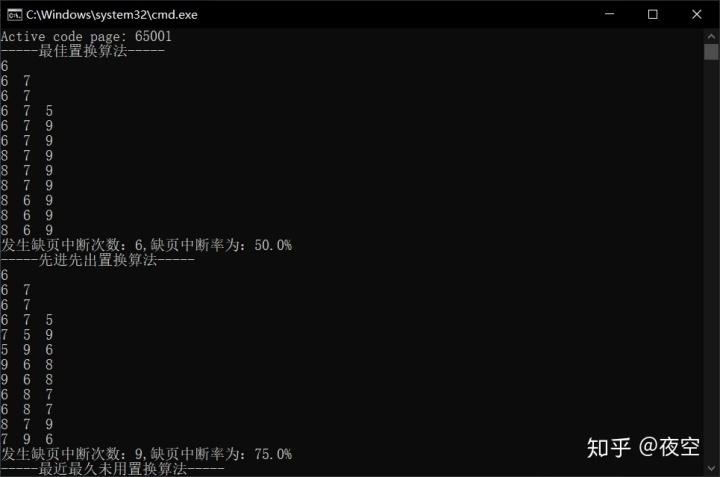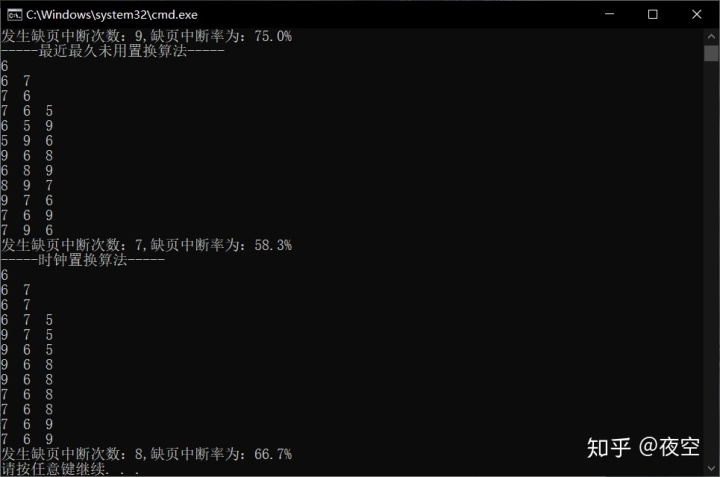背景图片来自舍友部分理论来自我学校的教科书
展开全文• 本次我要写的文章是操作系统的页面置换算法（OPT、FIFO、LRU、CLOCK）。页面置换算法不是很难，想必搜我这种文章的应该是都懂原理。所以在这里，简单概括算法特点，主要是展示代码。最佳置换算法——OPT（Optimal）...
• 页面置换算法: 资源包含三个算法：OPT---最佳置换算法、//FIFO---先进先出、//LRU---最近最久未使用 操作：用户输入物理块数、页面待要访问的个数、每个页面编号，计算出缺页数、置换数、缺页率 语言：C++ 运行环境...
• 操作系统 c++实现页面置换算法 功能基本完善 运行良好
• 利用C++语言来实现操作系统中的几种页面置换算法
• 该工程具体是在codeblock上面实现了操作系统课程上讲解的页面置换算法，包括先进先出（FIFO）、最佳置换算法（OPT）、最久最近未使用算法（LRU）。 具体实现功能有： 1、建立相应的数据结构 2、在屏幕上显示页面...
• 页面置换算法实验 本次实验一共用到了两个封装的类。 一个是作业的类，Block，其中的属性包括其中存入的页面的页号，和布尔类型的是否为空的属性。 另一个是pageRaplacing类，用来进行页面置换算法。 包括页面数组...操作系统
• 最佳置换算法 先来先服务置换算法 最近最久未使用置换算法 代码示例 #include<iostream> #include<algorithm> #include<array> #include<vector> using namespace std; class page_...操作系统 数据结构...

# 页面置换算法c++c++ 订阅